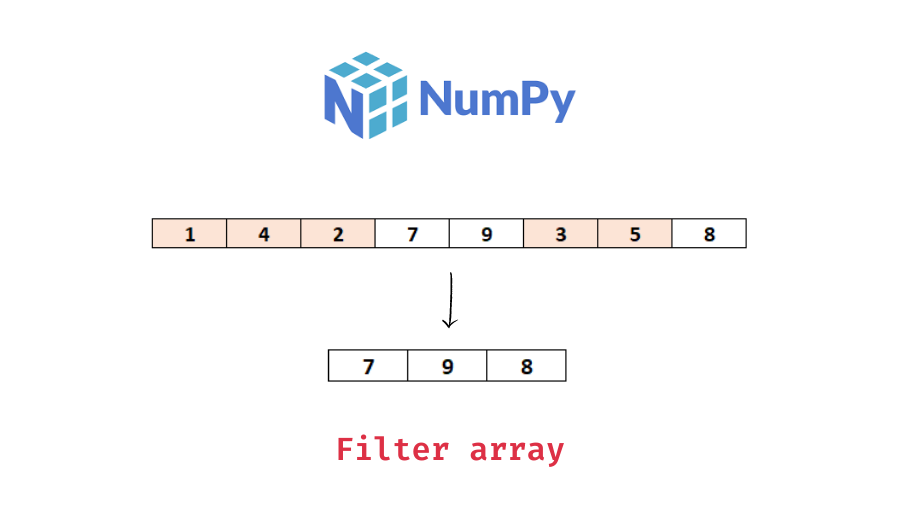# Filter a Numpy Array – With Examples

In this tutorial, we will look at how to filter a numpy array.

You can filter a numpy array by creating a list or an array of boolean values indicative of whether or not to keep the element in the corresponding array. This method is called boolean mask slicing. For example, if you filter the array `[1, 2, 3]` with the boolean list `[True, False, True]`, the filtered array would be `[1, 3]`.

The following is the syntax to filter a numpy array using this method –

```# arr is a numpy array
# boolean array of which elements to keep, here elements less than 4
# filter the array
# above filtering in a single line
arr_filtered = arr[arr < 4]```

Alternatively, you can also use `np.where()` to get the indexes of the elements to keep and filter the numpy array based on those indexes. The following is the syntax –

```# arr is a numpy array
# indexes to keep based on the condition, here elements less than 4
indexes_to_keep = np.where(arr < 4)
# filter the array
arr_filtered = arr[indexes_to_keep]
# above filtering in a single line
arr_filtered = arr[np.where(arr < 4)]```

Let’s look at some examples to better understand the usage of the above methods for different use-cases.

First, we will create a numpy array that we will be using throughout this tutorial –

```import numpy as np

# create a numpy array
arr = np.array([1, 4, 2, 7, 9, 3, 5, 8])
# print the array
print(arr)```

Output:

`[1 4 2 7 9 3 5 8]`

Let’s filter the above array `arr` on a single condition, say elements greater than 5 using the boolean masking method.

📚 Data Science Programs By Skill Level

Introductory

Intermediate ⭐⭐⭐

🔎 Find Data Science Programs 👨‍💻 111,889 already enrolled

Disclaimer: Data Science Parichay is reader supported. When you purchase a course through a link on this site, we may earn a small commission at no additional cost to you. Earned commissions help support this website and its team of writers.

```# boolean mask of elements to keep

# filter the array
# show the filtered array
print(arr_filtered)```

Output:

```[False False False  True  True False False  True]
[7 9 8]```

You can see that we printed the boolean mask and the filtered array. Masking and filtering can be done in a single line –

```# filter array
filtered_arr = arr[arr > 5]
print(filtered_arr)```

Output:

`[7 9 8]`

Let’s now go ahead and perform the same filtering, this time using `np.where()` instead of a boolean list or array.

```# indexes of elements to keep
indexes_to_keep = np.where(arr > 5)
print(indexes_to_keep)

# filter the array
arr_filtered = arr[indexes_to_keep]
# show the filtered array
print(arr_filtered)```

Output:

```(array([3, 4, 7], dtype=int64),)
[7 9 8]```

The indexes of elements to keep is printed followed by the filtered array. The np.where() function gives us the indexes satisfying the condition which are then used to filter the array. A shorter version of the above code is –

```# filter array
filtered_arr = arr[np.where(arr > 5)]
print(filtered_arr)```

Output:

`[7 9 8]`

To filter the array on multiple conditions, you can combine the conditions together using parenthesis `()` and the “and” `&` operator – `((condition1) & (condition2) & ...)`

Let’s filter the array “arr” on two conditions – greater than 5 and less than 9 using boolean masking.

```# filter array
filtered_arr = arr[(arr > 5) & (arr < 9)]
print(filtered_arr)```

Output:

`[7 8]`

The returned array only contains elements from the original array that are greater than 5 and less than 9, satisfying both the conditions.

Let’s now perform the same filtering using `np.where()`

```# filter array
filtered_arr = arr[np.where(((arr > 5) & (arr < 9)))]
print(filtered_arr)```

Output:

`[7 8]`

We get the same result satisfying both the conditions.

In the above examples, we filtered the array on two conditions but this method can easily be extended to multiple conditions.

With this, we come to the end of this tutorial. The code examples and results presented in this tutorial have been implemented in a Jupyter Notebook with a python (version 3.8.3) kernel having numpy version 1.18.5

•